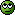# Maths Notation (Sets)

#### demon8991

Soldato
Just trying to get my head round some maths notation some of it is ok but some im stuggling with.

Is {3} a sub set of {3}?

Does {3} = {{3}}?

Does 0 belong to the empty set?

Does 0 belong to the empty set inside a set?

Im also struggling with this double sum question, as im trying to teach myself this subject.Soldato
I think so
I'm not sure
Definately not
I don't think so

#### demon8991

Soldato
OP
Yer i know mate, im finding it really hard to get my head round.

#### Lagz

Associate
demon8991 said:
Is {3} a sub set of {3}?

Does {3} = {{3}}?

Does 0 belong to the empty set?

Does 0 belong to the empty set inside a set?

Yes - but not a proper subset
No
What? No
What? No

Whats the actual question for that sum?

#### demon8991

Soldato
OP
Just got to evaluate the double sum.

#### neocon

Soldato
WHATS THE POINT OF KNOWING THIS?

If you cant easily work it out, quit.

#### Lagz

Associate
neocon said:
WHATS THE POINT OF KNOWING THIS?

If you cant easily work it out, quit.Just got to evaluate the double sum.

All you need to know is that 1+2+....+n = 0.5n(n+1)

Then you can get rid of the sums. Seems a very strange question though?!

#### demon8991

Soldato
OP

So the answer is just 0.5(n+1)?

#### DaveF

Associate
demon8991 said:

So the answer is just 0.5(n+1)?
No. If you look at the inner sum, you have (1+i) + (2 + i) + ... + (i + i). Split this up as 1 + 2 + ... + i, and i + i + ... + i (each sum has i terms). Use Lagz comment to work out 1 + 2 + ... + i and your own common sense for the other one. Then once you've worked out what the inner sum comes to (it will be a function of i), use that to work out what the outer sum is.

Hint: You will probably find it helpful to know that 1 + 4 + 9 + 16 + ... + n^2 = n(n+1)(2n+1)/6

#### demon8991

Soldato
OP
Therefore does the inner sum equal i^2 + i + 1?

#### DaveF

Associate
demon8991 said:
Therefore does the inner sum equal i^2 + i + 1?
No. How did you work that out?

Whatever answer you get, it makes sense to check it for small values of i. Your answer doesn't work when i = 1.

#### demon8991

Soldato
OP
Oh god im completly confused, i really need to be able to do this and its driving me nuts that i cant (im one of those people).

Suspended
demon8991 said:
Oh god im completly confused, i really need to be able to do this and its driving me nuts that i cant (im one of those people).
You want to evaluate the two sums completely separately. If you do the sum over j first, remember that i isn't being summed over at all, so what you have is:

(i+1) + (i+2) + (i+3) + ... + (i+i)

so you're adding i together i times, and then you're adding all the numbers from 1 to i. That should give you an expression for the sum in brackets, and then you need to sum that over i as well. To do that you'll need to know the expression for the sum from i=1 to n of i^2... which you can either work out or google.

Edit: Hah, I realised that I just repeated DaveF's post.

To clarify one of your earlier questions, the reason that {3} is not the same as {{3}} is because sets are only equal when every element is the same. The only element of the first set is 3, while the only element of the second set is {3}. Are these equal? Clearly not, because one is a number and the other is a set.

Last edited:

#### iCraig

Permabanned
neocon said:
WHATS THE POINT OF KNOWING THIS?

To learn it and understand it.

Although I see where you're coming from in a way. Knowledge is useless without application, but if he wants to learn it, or needs to learn it, then that's application enough.